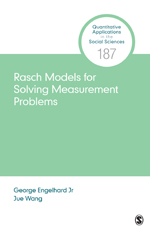# Rasch Models for Solving Measurement Problems: Invariant Measurement in the Social Sciences

This book introduces current perspectives on Rasch measurement theory with an emphasis on developing Rasch-based scales. Rasch measurement theory represents a paradigm shift in measurement theory away from classical test theory and creates a framework for scaling that can yield invariant measurement. Rasch Models for Solving Measurement Problems: Invariant Measurement in the Social Sciences is a broadly accessible text. Authors George Engelhard Jr and Jue Wang introduce Rasch measurement theory step by step, with chapters on scale construction, evaluation, maintenance, and use. Points are illustrated and techniques are demonstrated through an extended example: The Food Insecurity Experience (FIE) Scale. The Rasch analyses in the book are run using the Facets computer program. Facets syntax, and R code for the ERMA program created by the authors to obtain parameter estimates and to examine model-data fit, together with sample data sets are all available on a website for the book.

•••